Select Page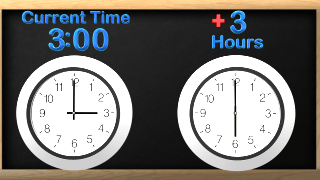#### Elapsed Time

Our video on elapsed time teaches how to know how much time has passed or will pass. Follow along and pause at any time to have kids figure it out on their own clocks.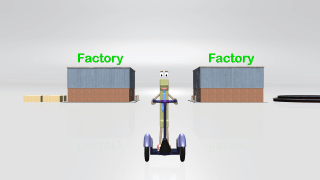#### Multiplication Vocabulary

Students will learn the vocabulary behind multiplication in this fun video for kids. This is a great introduction to multiplication that helps students understand the words that are used in multiplication.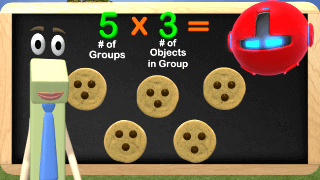#### Multiplication Relations

Students will learn about Multiplication for 3rd grade in this fun video. We help kids see relations between number of groups and number of objects in each group. Pause the video at any time to let students answer the questions.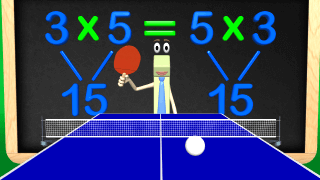#### Commutative Multiplication

While playing Ping Pong we learn about the Commutative Property of Multiplication for 3rd Grade. Join in the video with us by following along and you can even play ping pong with us! Can numbers be switched around when multiplying?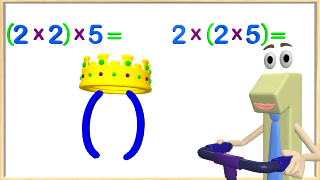#### Associative Property of Multiplication

Come learn about the Associative Property of Multiplication with King Parenthesis and the Number One. We learn about multiplication and changing the order in where you start does not matter. You still start with the King First!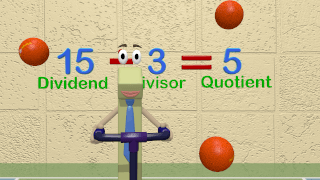Come learn the basic vocabulary for Division for 3rd grade! This fun math video engages students and helps teachers start out the subject of Division. Feel free to pause the video at any time to explain or answer questions. This is great to review concepts.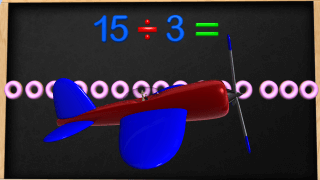#### Division Basics

Learn the basics of division in this fun video where we learn different ways to divide. We use number lines, grouping of objects and repeated subtraction. Feel free to pause the division video to challenge the kids in class.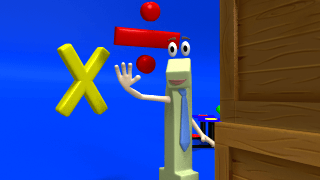#### Multiplication and Division Relationships

Come play tag with us as we learn about the relationship between multiplication and division. Working with division can be tough but so much easier as we understand how multiplication can help us. Join us in this fun math video for kids.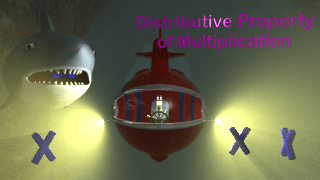#### Distributive Property of Multiplication

Watch our Awesome Video on Distributive Property of Multiplication. Watch out for sharks as we find how using the distributive property can help us solve multiplication problems. This skill is awesome….. and so are the sharks!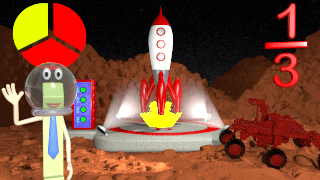Come help the Number One recharge the batteries in his rocket ship so that he can get home. The rocket ship only takes fractions like 1/b. If you don’t know what this is watch it and learn with us!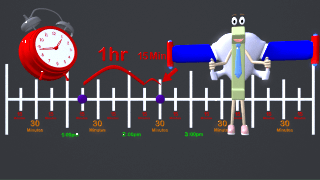Come learn how to use a number line to add and subtract time! Join us in the clock classroom and lets learn how to solve addition and subtraction time word problems.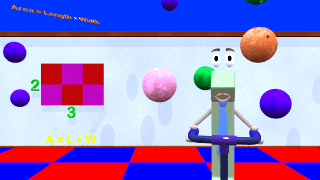#### Area of Squares and Rectangles

This video teaches how to find area of a square or rectangle. We learn the basics of area and jump right into the FUN! Feel free to pause anytime to help kids understand it better.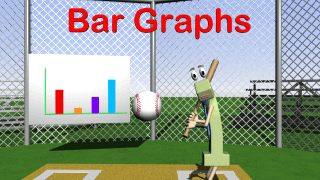#### Bar Graph Problems 3rd Grade

Come learn all about solving problems with Bar Graphs in this fun video for 3rd Grade. We solve one and two step word problems in this video.  See if you can strike out the Number One!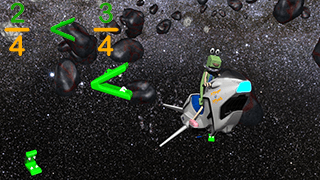#### Comparing Fractions

Help me avoid the Space Gators by comparing fractions in this fun math video. We teach how to compare fractions for 3rd grade by using models and understanding differences in numerator and denominator. Pause the video at any time to answer questions.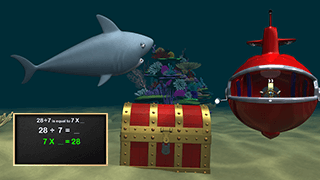#### Division with Unknown Factors

Come to the Division with Unknown Factor Reef and learn how multiplication can help you solve division problems. Using factor families or trees can help with solving multiplication and division problems. Come learn with us!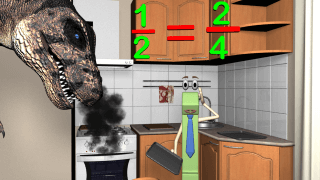#### Equivalent Fractions

Learn about Equivalent Fractions while the Number One keeps his T Rex friend happy. Kids will learn about equivalent fractions and whole numbers expressed as fractions in this fun math video. Enjoy and pause it at any time to answer questions.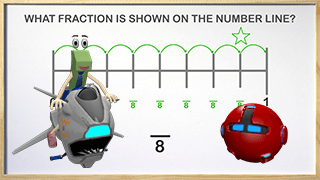#### Fractions on a Number Line

Come learn about Fractions on a Number Line in our fun Race! Mr. Robot is helping us learn fractions and how they appear on a number line. Using a number line is a great way to teach how fractions work. Enjoy and pause it if needed.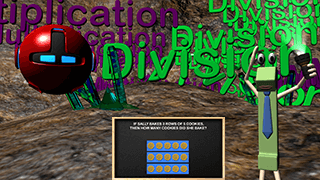#### Multiplication Division Word Problems

Kids will learn how to solve word problems involving Multiplication and Division for 3rd Grade. We use arrays and models to help kids solve the problems. Try to be quiet in the caves!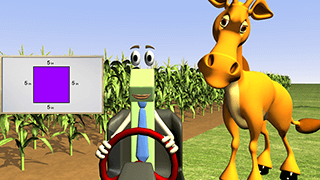#### Perimeter of Polygons

Come learn all about Perimeter of Polygons in this fun video for Kids. Help the Number One find the perimeter of his corn field and see how perimeter and area are different.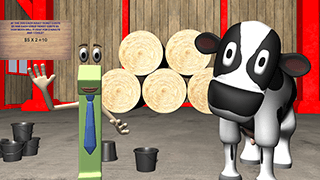#### Solve Two Step Word Problems

Join us on the farm as we solve Two Step word problems. Many word problems require more than one step to solve so come learn with us.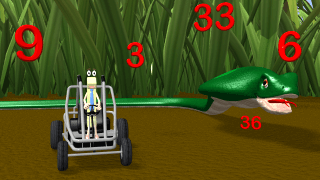#### 3 Times Table Video

Learn the Three Times Tables with Mr. Snake and the Number One in this fun video. We count by 3 and then multiply by 3. This video is great for helping to memorize times tables.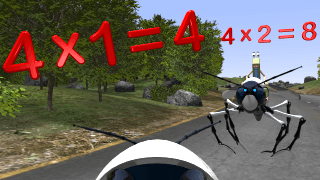#### 4 Times Table

Come fly with our Robot wasp as we explore the 4 times table in this fun video. This video is great for introducing the 4 times table to the students. It can be a fun way to start the math portion of your class.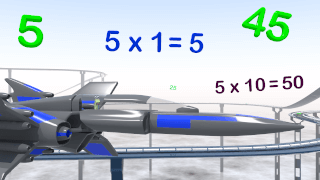#### 5 Times Table

Join the Race as we learn the 5 Times Table and count by 5’s. This video is great for kids learning to either count by 5’s or learning the 5 multiplication tables. Enjoy!

### Here are some videos that we thought might be useful in Third Grade but might be found in other grades.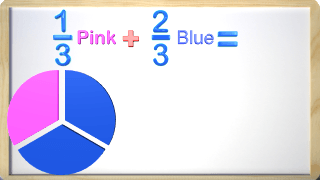This video is in our 4th grade but sometimes teachers use it in 3rd as well.#### Controlling Emotions

Watch this video in class to help kids know how to control emotions.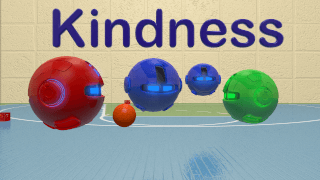#### Kindness Video for Kids

This is a video we created to help kids learn kindness and self worth. It was designed for our youtube channel but we post all our videos here without ads. We hope kids learn that we are all different and that is what makes us amazing!